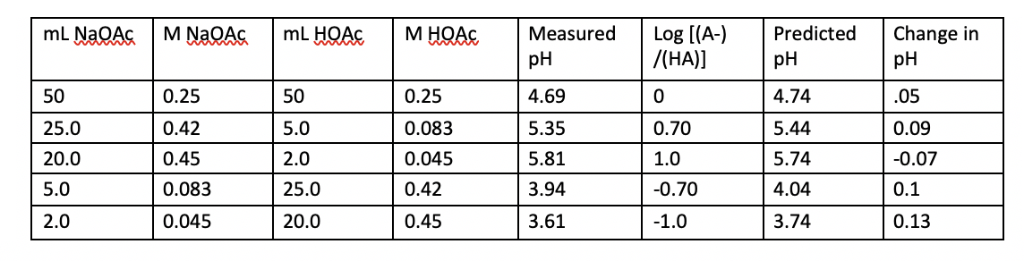Homework Help Question & Answers

ML NaQAGM NaQAG mL HOA M HQAcMeasuredLog [(A-) Predicted Change in /НА)) рН 4.69 5.35 5.81 3.94 3...

Does this data fit the Henderson-Hasselbach equation?mL NaQAGM NaQAG mL HOA M HQAcMeasuredLog [(A-) Predicted Change in /НА)) рН 4.69 5.35 5.81 3.94 3.61 pH 4.74 5.44 5.74 4.04 3.74 pH .05 0.09 0.07 0.1 0.13 0.25 0.083 0.045 0.42 0.45 50 25.0 20.0 5.0 2.0 0.25 0.42 0.45 0.083 0.045 50 5.0 2.0 25.0 20.0 0.70 1.0 0.70 1.0

Handerson eqn.is

pH = pKa + log [A-]/[HA], here pka values is not given so the data cannot be fit in this eqn.

Add Answer of: ML NaQAGM NaQAG mL HOA M HQAcMeasuredLog [(A-) Predicted Change in /НА)) рН 4.69 5.35 5.81 3.94 3...
More Homework Help Questions Additional questions in this topic.

• The demand equation for a certain type of printer is given by: d=-200p+35,000 The supply equation is predicted to be s=p squared + 400p-20,000 find the equilibrium price

Need Online Homework Help?

Get FREE EXPERT Answers
WITHIN MINUTES
Related Questions